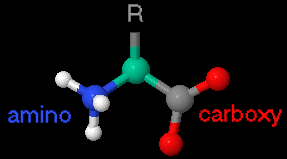Directions

[Back to OMM Exhibits]

## Notes: 1) loading of all molecules may take some time; 2) molecules are not all shown at the same scale

### Backbones are oriented amino (left) to carboxy (right)### Name colors indicate:hydrophobic,polar (non-charged),polar (charged-basic),polar (charged-acidic)

 alanine (ala = A) rotate 360o valine (val = V) rotate 360o leucine (leu = L) rotate 360o isoleucine (ile = I) rotate 360o methionine (met = M) rotate 360o proline (pro = P) rotate 360o phenylalanine (phe = F) rotate 360o tryptophan (trp = W) rotate 360o glycine (gly = G) rotate 360o serine (ser = S) rotate 360o threonine (thr = T) rotate 360o glutamine (gln = Q) rotate 360o asparagine (asn = N) rotate 360o cysteine (cys = C) rotate 360o tyrosine (tyr = Y) rotate 360o glutamic acid (glu = E) rotate 360o aspartic acid (asp = D) rotate 360o arginine (arg = R) rotate 360o lysine (lys = K) rotate 360o histidine (his = H) rotate 360o